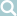绑定 this 的三种方法

this 的动态切换，固然为 JavaScript 创造了巨大的灵活性，但也使得编程变得困难和模糊。有时，需要把 this 固定下来，避免出现意想不到的情况。JavaScript 提供了 call、apply、bind 这三个方法，来切换/固定 this 的指向。

this 是什么？

var A = {
name: '张三',
describe: function() {
return '姓名：' + this.name
}
}

var B = {
name: '李四'
}

B.describe = A.describe
B.describe()
// "姓名：李四"

function f() {
return '姓名：' + this.name
}

var A = {
name: '张三',
describe: f
}

var B = {
name: '李四',
describe: f
}

A.describe() // "姓名：张三"
B.describe() // "姓名：李四"

var A = {
name: '张三',
describe: function() {
return '姓名：' + this.name
}
}

var name = '李四'
var f = A.describe
f() // "姓名：李四"

Function.prototype.call()

var obj = {}

var f = function() {
return this
}

f() === window // true
f.call(obj) === obj // true

call 方法的参数，应该是一个对象。如果参数为空、null 和 undefined，则默认传入全局对象。

var n = 123
var obj = { n: 456 }

function a() {
console.log(this.n)
}

a.call() // 123
a.call(null) // 123
a.call(undefined) // 123
a.call(window) // 123
a.call(obj) // 456

var f = function() {
return this
}

f.call(5)
// Number {[[PrimitiveValue]]: 5}

call 方法还可以接受多个参数。

func.call(thisValue, arg1, arg2, ...)

call 的第一个参数就是 this 所要指向的那个对象，后面的参数则是函数调用时所需的参数。

function add(a, b) {
return a + b
}

add.call(this, 1, 2) // 3

Function.prototype.apply()

apply 方法的作用与 call 方法类似，也是改变 this 指向，然后再调用该函数。唯一的区别就是，它接收一个数组作为函数执行时的参数，使用格式如下。

func.apply(thisValue, [arg1, arg2, ...])

apply 方法的第一个参数也是 this 所要指向的那个对象，如果设为 null 或 undefined，则等同于指定全局对象。第二个参数则是一个数组，该数组的所有成员依次作为参数，传入原函数。原函数的参数，在 call 方法中必须一个个添加，但是在 apply 方法中，必须以数组形式添加。

function f(x, y) {
console.log(x + y)
}

f.call(null, 1, 1) // 2
f.apply(null, [1, 1]) // 2

Function.prototype.bind()

bind 方法用于将函数体内的 this 绑定到某个对象，然后返回一个新函数。

var d = new Date()
d.getTime() // 1481869925657

var print = d.getTime
print() // Uncaught TypeError: this is not a Date object.

bind 方法可以解决这个问题。

var print = d.getTime.bind(d)
print() // 1481869925657

bind 方法的参数就是所要绑定 this 的对象，下面是一个更清晰的例子。

var counter = {
count: 0,
inc: function() {
this.count++
}
}

var func = counter.inc.bind(counter)
func()
counter.count // 1

this 绑定到其他对象也是可以的。

var counter = {
count: 0,
inc: function() {
this.count++
}
}

var obj = {
count: 100
}
var func = counter.inc.bind(obj)
func()
obj.count // 101

bind 还可以接受更多的参数，将这些参数绑定原函数的参数。

var add = function(x, y) {
return x * this.m + y * this.n
}

var obj = {
m: 2,
n: 2
}